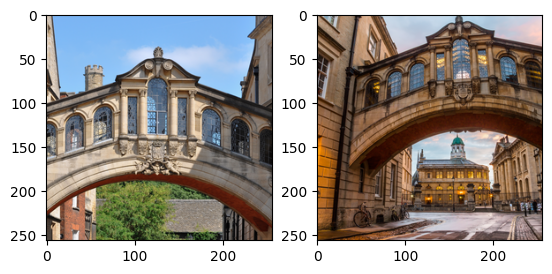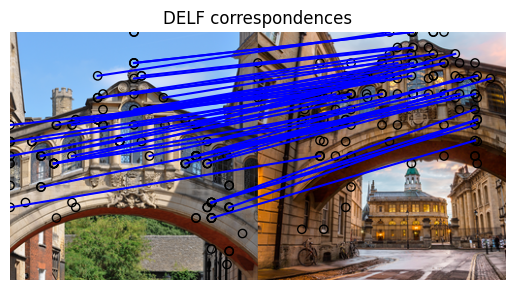Help protect the Great Barrier Reef with TensorFlow on Kaggle

如何使用 DELF 和 TensorFlow Hub 匹配图像

TensorFlow Hub (TF-Hub) 是一个分享打包在可重用资源（尤其是预训练的模块）中的机器学习专业知识的平台。

设置

pip install scikit-image
from absl import logging

import matplotlib.pyplot as plt
import numpy as np
from PIL import Image, ImageOps
from scipy.spatial import cKDTree
from skimage.feature import plot_matches
from skimage.measure import ransac
from skimage.transform import AffineTransform
from six import BytesIO

import tensorflow as tf

import tensorflow_hub as hub
from six.moves.urllib.request import urlopen

数据

Choose images

path = tf.keras.utils.get_file(url.split('/')[-1], url)
image = Image.open(path)
image = ImageOps.fit(image, (new_width, new_height), Image.ANTIALIAS)
return image

plt.subplot(1,2,1)
plt.imshow(image1)
plt.subplot(1,2,2)
plt.imshow(image2)
7020544/7013850 [==============================] - 0s 0us/step
7028736/7013850 [==============================] - 0s 0us/step
14172160/14164194 [==============================] - 1s 0us/step
14180352/14164194 [==============================] - 1s 0us/step
<matplotlib.image.AxesImage at 0x7f1e0840d7d0>将 DELF 模块应用到数据

DELF 模块使用一个图像作为输入，并使用向量描述需要注意的点。以下单元包含此 Colab 逻辑的核心。

2021-08-13 20:58:30.461177: I tensorflow/stream_executor/cuda/cuda_gpu_executor.cc:937] successful NUMA node read from SysFS had negative value (-1), but there must be at least one NUMA node, so returning NUMA node zero
2021-08-13 20:58:30.470011: I tensorflow/stream_executor/cuda/cuda_gpu_executor.cc:937] successful NUMA node read from SysFS had negative value (-1), but there must be at least one NUMA node, so returning NUMA node zero
2021-08-13 20:58:30.470910: I tensorflow/stream_executor/cuda/cuda_gpu_executor.cc:937] successful NUMA node read from SysFS had negative value (-1), but there must be at least one NUMA node, so returning NUMA node zero
2021-08-13 20:58:30.472676: I tensorflow/core/platform/cpu_feature_guard.cc:142] This TensorFlow binary is optimized with oneAPI Deep Neural Network Library (oneDNN) to use the following CPU instructions in performance-critical operations:  AVX2 AVX512F FMA
To enable them in other operations, rebuild TensorFlow with the appropriate compiler flags.
2021-08-13 20:58:30.473243: I tensorflow/stream_executor/cuda/cuda_gpu_executor.cc:937] successful NUMA node read from SysFS had negative value (-1), but there must be at least one NUMA node, so returning NUMA node zero
2021-08-13 20:58:30.474250: I tensorflow/stream_executor/cuda/cuda_gpu_executor.cc:937] successful NUMA node read from SysFS had negative value (-1), but there must be at least one NUMA node, so returning NUMA node zero
2021-08-13 20:58:30.475211: I tensorflow/stream_executor/cuda/cuda_gpu_executor.cc:937] successful NUMA node read from SysFS had negative value (-1), but there must be at least one NUMA node, so returning NUMA node zero
2021-08-13 20:58:31.055466: I tensorflow/stream_executor/cuda/cuda_gpu_executor.cc:937] successful NUMA node read from SysFS had negative value (-1), but there must be at least one NUMA node, so returning NUMA node zero
2021-08-13 20:58:31.056468: I tensorflow/stream_executor/cuda/cuda_gpu_executor.cc:937] successful NUMA node read from SysFS had negative value (-1), but there must be at least one NUMA node, so returning NUMA node zero
2021-08-13 20:58:31.057360: I tensorflow/stream_executor/cuda/cuda_gpu_executor.cc:937] successful NUMA node read from SysFS had negative value (-1), but there must be at least one NUMA node, so returning NUMA node zero
2021-08-13 20:58:31.058225: I tensorflow/core/common_runtime/gpu/gpu_device.cc:1510] Created device /job:localhost/replica:0/task:0/device:GPU:0 with 14648 MB memory:  -> device: 0, name: Tesla V100-SXM2-16GB, pci bus id: 0000:00:05.0, compute capability: 7.0
2021-08-13 20:58:32.092480: I tensorflow/compiler/mlir/mlir_graph_optimization_pass.cc:185] None of the MLIR Optimization Passes are enabled (registered 2)
def run_delf(image):
np_image = np.array(image)
float_image = tf.image.convert_image_dtype(np_image, tf.float32)

return delf(
image=float_image,
score_threshold=tf.constant(100.0),
image_scales=tf.constant([0.25, 0.3536, 0.5, 0.7071, 1.0, 1.4142, 2.0]),
max_feature_num=tf.constant(1000))
result1 = run_delf(image1)
result2 = run_delf(image2)
2021-08-13 20:58:35.697132: I tensorflow/stream_executor/cuda/cuda_dnn.cc:369] Loaded cuDNN version 8100
2021-08-13 20:58:36.262184: I tensorflow/core/platform/default/subprocess.cc:304] Start cannot spawn child process: No such file or directory

使用位置和描述向量匹配图像

TensorFlow is not needed for this post-processing and visualization

def match_images(image1, image2, result1, result2):
distance_threshold = 0.8

num_features_1 = result1['locations'].shape
print("Loaded image 1's %d features" % num_features_1)

num_features_2 = result2['locations'].shape
print("Loaded image 2's %d features" % num_features_2)

# Find nearest-neighbor matches using a KD tree.
d1_tree = cKDTree(result1['descriptors'])
_, indices = d1_tree.query(
result2['descriptors'],
distance_upper_bound=distance_threshold)

# Select feature locations for putative matches.
locations_2_to_use = np.array([
result2['locations'][i,]
for i in range(num_features_2)
if indices[i] != num_features_1
])
locations_1_to_use = np.array([
result1['locations'][indices[i],]
for i in range(num_features_2)
if indices[i] != num_features_1
])

# Perform geometric verification using RANSAC.
_, inliers = ransac(
(locations_1_to_use, locations_2_to_use),
AffineTransform,
min_samples=3,
residual_threshold=20,
max_trials=1000)

print('Found %d inliers' % sum(inliers))

# Visualize correspondences.
_, ax = plt.subplots()
inlier_idxs = np.nonzero(inliers)
plot_matches(
ax,
image1,
image2,
locations_1_to_use,
locations_2_to_use,
np.column_stack((inlier_idxs, inlier_idxs)),
matches_color='b')
ax.axis('off')
ax.set_title('DELF correspondences')
match_images(image1, image2, result1, result2)